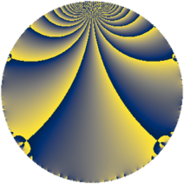Properties

 Label 342.3.tLevel $342$ Weight $3$ Character orbit 342.t Rep. character $\chi_{342}(31,\cdot)$ Character field $\Q(\zeta_{6})$ Dimension $80$ Newform subspaces $1$ Sturm bound $180$ Trace bound $0$

Related objects

Defining parameters

 Level: $$N$$ $$=$$ $$342 = 2 \cdot 3^{2} \cdot 19$$ Weight: $$k$$ $$=$$ $$3$$ Character orbit: $$[\chi]$$ $$=$$ 342.t (of order $$6$$ and degree $$2$$) Character conductor: $$\operatorname{cond}(\chi)$$ $$=$$ $$171$$ Character field: $$\Q(\zeta_{6})$$ Newform subspaces: $$1$$ Sturm bound: $$180$$ Trace bound: $$0$$

Dimensions

The following table gives the dimensions of various subspaces of $$M_{3}(342, [\chi])$$.

Total New Old
Modular forms 248 80 168
Cusp forms 232 80 152
Eisenstein series 16 0 16

Trace form

 $$80 q - 6 q^{3} + 80 q^{4} - 4 q^{6} + 2 q^{7} - 2 q^{9} + O(q^{10})$$ $$80 q - 6 q^{3} + 80 q^{4} - 4 q^{6} + 2 q^{7} - 2 q^{9} - 6 q^{11} + 30 q^{13} + 24 q^{15} - 160 q^{16} + 6 q^{17} + 22 q^{19} + 24 q^{23} + 8 q^{24} + 400 q^{25} - 126 q^{27} - 4 q^{28} - 20 q^{30} + 24 q^{31} + 30 q^{33} + 108 q^{35} + 4 q^{36} + 24 q^{38} + 6 q^{39} - 8 q^{42} + 76 q^{43} + 12 q^{44} + 296 q^{45} + 168 q^{47} + 24 q^{48} - 306 q^{49} + 144 q^{50} + 234 q^{51} + 60 q^{52} + 72 q^{53} - 28 q^{54} - 90 q^{57} + 36 q^{60} - 28 q^{61} - 36 q^{62} + 68 q^{63} - 640 q^{64} + 288 q^{65} - 8 q^{66} - 336 q^{67} - 12 q^{68} - 48 q^{69} + 162 q^{71} - 48 q^{72} - 92 q^{73} - 24 q^{74} - 732 q^{75} + 4 q^{76} + 228 q^{77} - 372 q^{78} + 102 q^{79} + 130 q^{81} - 48 q^{82} + 78 q^{83} - 984 q^{87} + 72 q^{88} + 216 q^{89} - 456 q^{90} + 96 q^{91} - 48 q^{92} + 24 q^{93} + 720 q^{95} + 32 q^{96} + 90 q^{97} + 216 q^{98} - 40 q^{99} + O(q^{100})$$

Decomposition of $$S_{3}^{\mathrm{new}}(342, [\chi])$$ into newform subspaces

Label Dim. $$A$$ Field CM Traces $q$-expansion
$a_{2}$ $a_{3}$ $a_{5}$ $a_{7}$
342.3.t.a $80$ $9.319$ None $$0$$ $$-6$$ $$0$$ $$2$$

Decomposition of $$S_{3}^{\mathrm{old}}(342, [\chi])$$ into lower level spaces

$$S_{3}^{\mathrm{old}}(342, [\chi]) \cong$$ $$S_{3}^{\mathrm{new}}(171, [\chi])$$$$^{\oplus 2}$$